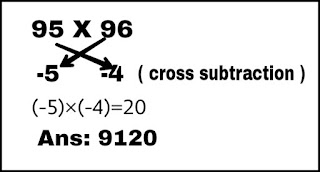Maths short Tricks

Hey dear, In this blog I am going to tell you about some short math tricks which will help you to manage time during your competitive examination.

1: Divisibility by denominator  5 (xxxx/5)
Example:   234/5 = ?
Let us solve this with short trick-
step1: multiply numerator by 2 ( e.g. 234 X 2 = 468 )
step2: put decimal(.) after first digit from RHS. ( 46.8)
Ans: 46.8 is it correct? Let us come into next trick-

2: Divisibility by denominator 25 (xxx/25)
Example:  456/25 = ?
step1: multiply numerator by 4 ( e.g. 456 X 4 = 1824 )
step2: put decimal(.) after second digit from RHS. ( 18.24 )
Ans: 18.24 is it correct?

3: Divisibility by denominator 125 (xxx/125)
Example:  3453/125 = ?
Step1: multiply numerator by 8 ( e.g. 3453 X 8 = 27624 )
Step2: put decimal(.) after third digit from RHS ( 27.624 )
Ans: 27.624 is it correct? Now let us come to some short multiplication Trick.

Multiplication by short Trick:

1: Multiplication of two numbers less than 100.
Example:      95 X 96
Step1:  Subtract both numbers from 100.
100-95= 5  & 100 – 96 = 4
Step2:  Subtract  4 from 95 or 5 from 96. The value will be same, i.e, 91.
Step3:  Now multiply 4 and 5 i.e, 4x5=20.
Step4: Now  put  91 at LHS and 20 at RHS i.e, . Your answer will be 9120. Is it correct?
Lets go to another Trick.Short multiplication trick

2. Multiplication of two numbers more than 100.
Example:      103 X 106
Step1: Subtract 100 from both numbers.
103-100=3  &  106-100=6
Step2: Add 6 with 103 or 3 with 106. The value will be same in both case i.e, 109.
Step3: Now multiply 3 and 6 i.e, 3x6=18
Step4: Same as above step. Put 109 at LHS and 18 at RHS i.e, . Your answer will be 10918. Is it correct? Now let us come to a special case-Short Multiplication trick

3. Multiplication of two numbers, one is less than 100 and another is greater than 100.
Example:   97 X 102
Step1:  Subtract 97 from 100 and 100 from 102.
100-97=3  &  102-100=2
Step2:  Add 2 with 97 or Subtract  3 from 102. The value will be same i.e, 99.
Step 3: Multiply 3 and 2 i.e, 2x3=6
Step4: ( Little bit different from previous method ) Put 99 at LHS and 00 at RHS. i.e,  or this will be 9900.Short multiplication trick

These short tricks are seems to be long but after practicing one or two problems it will be easier and less time consuming. You will be able to do it quickly without writing any calculation.
Note: Main part i.e, in second step is to find difference between 100 and the given number. If it less than 100 put minus sign and if it is greater than 100 put plus sign as shown in above picture.
You may also search for  Quickest method to solve a equation visit here

1.2.Wow! Very impressive maths tricks. I have always maths phobia and solving maths problem in exams. I will definitly use these maths tricks during my exam. I have get some extra math solving ideas from Smile Tutor which is best learning platform for students.